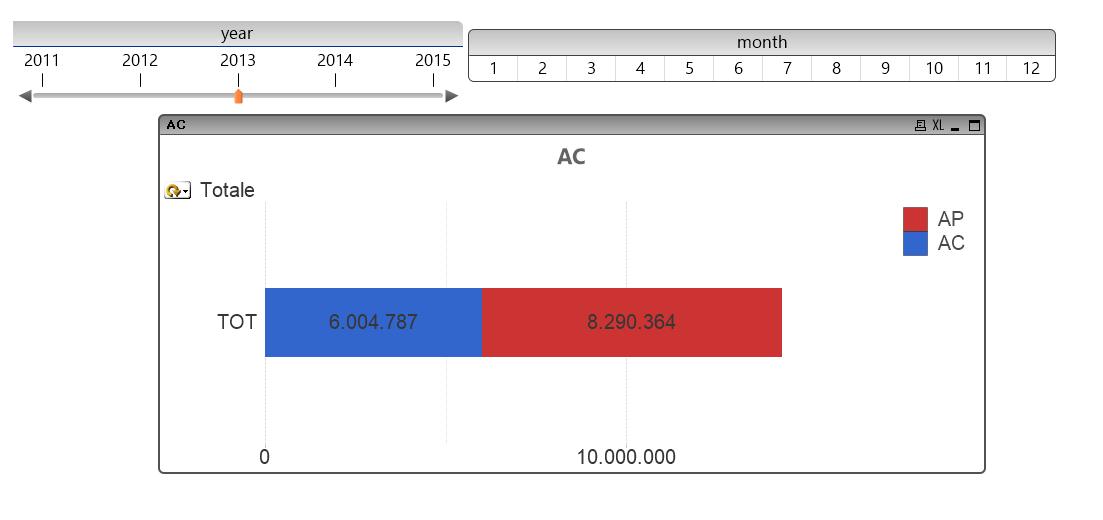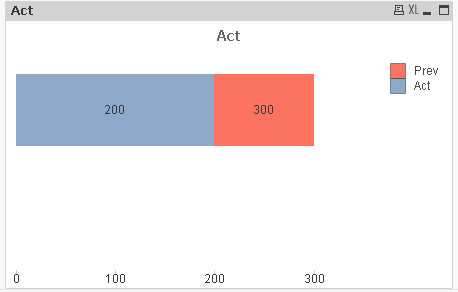# QlikView Layout & Visualizations

Discussion Board for collaboration on QlikView Layout & Visualizations.

Announcements
The #1 reason QlikView customers adopt Qlik Sense is a desire for a modern BI experience. Read More
cancel
Showing results for
Did you mean:
HighlightedContributor

## Overlap expressions in bar chart

Hello everybody,

I have the following 2 expressions, summing quantities for this year and last year:

Expression 1 (AC): Sum( {<Year = {(\$(=vYear)}, Month =>}

Expression 2 (AP) : Sum( {<Year = {(\$(=vYear-1)}>}

What I want to create is a bar chart where the 2 expression overlap, like in an actual vs budget scenario: AP is like the budget while AC is the actual.

As you can see from the picture, the problem is that, using stacked bar chart, the total length of the bar is the sum of the 2 expressions (6.004.787+8.290.364) because AP starts from the end of AC, whereas I want AP to start from 0 so that the total length is not the sum of the 2 expression.

Anyone knows a possible solution?

FrancescoTags (1)
1 Solution

Accepted Solutions
HighlightedMVP & Luminary

## Re: Overlap expressions in bar chart

Use as second expression Sum( {<Year = {(\$(=vYear-1)}>} ...) - Sum( {<Year = {(\$(=vYear)}, Month =>} ...)

talk is cheap, supply exceeds demand
8 Replies
HighlightedMVP & Luminary

## Re: Overlap expressions in bar chart

Use as second expression Sum( {<Year = {(\$(=vYear-1)}>} ...) - Sum( {<Year = {(\$(=vYear)}, Month =>} ...)

talk is cheap, supply exceeds demand
HighlightedSpecialist III

## Re: Overlap expressions in bar chart

Hi Francesco,

Use dual function.

Dual(ValueToShow with Format, ValueOfData).

First parameter is the value to show in graph, and second parameter is the lenght of your bar.

Regards!

HighlightedPartner

## Re: Overlap expressions in bar chart

Hi Francesco,

Adding to Gysbert & Manuel, I would use the Dual function too but as AP could be either greater than or less than AC, you would need to do something like this:

AC

if(Sum( {<Year = {(\$(=vYear)}, Month =>} YourValue) < Sum( {<Year = {(\$(=vYear-1)}, Month =>} YourValue),

Dual(

Sum( {<Year = {(\$(=vYear)}, Month =>} YourValue), //data to display in bar

Sum( {<Year = {(\$(=vYear)}, Month =>} YourValue) //length of bar

),

if(Sum( {<Year = {(\$(=vYear)}, Month =>} YourValue) >= Sum( {<Year = {(\$(=vYear-1)}, Month =>} YourValue),

Dual(

Sum( {<Year = {(\$(=vYear)}, Month =>} YourValue), //data to display in bar

Sum( {<Year = {(\$(=vYear-1)}, Month =>} YourValue) - Sum( {<Year = {(\$(=vYear)}, Month =>} YourValue) //length of bar

)

))

AP

if(Sum( {<Year = {(\$(=vYear-1)}, Month =>} YourValue) < Sum( {<Year = {(\$(=vYear)}, Month =>} YourValue),

Dual(

Sum( {<Year = {(\$(=vYear-1)}, Month =>} YourValue), //data to display in bar

Sum( {<Year = {(\$(=vYear)}, Month =>} YourValue)-Sum( {<Year = {(\$(=vYear-1)}, Month =>} YourValue) //length of bar

),

if(Sum( {<Year = {(\$(=vYear-1)}, Month =>} YourValue) >= Sum( {<Year = {(\$(=vYear)}, Month =>} YourValue),

Dual(

Sum( {<Year = {(\$(=vYear-1)}, Month =>} YourValue), //data to display in bar

Sum( {<Year = {(\$(=vYear-1)}, Month =>} YourValue) - Sum( {<Year = {(\$(=vYear)}, Month =>} YourValue) //length of bar

)

))

Then you should colour code the background of AP to some thing like:

if(Sum( {<Year = {(\$(=vYear)}, Month =>} YourValue) < Sum( {<Year = {(\$(=vYear-1)}, Month =>} YourValue),

LightRed(),

LightGreen()

)

This all should keep the total size of the bar at the largest amount (either AC or AP) and AP will be Red if it is greater than last year and Green if it is lower.

HighlightedSpecialist III

## Re: Overlap expressions in bar chart

Hi Francesco,

See this example:Act = Sum( {<Year = {(\$(=vYear)}, Month =>} YourFieldValue)

Prev = Dual(Num(Sum( {<Year = {'\$(=vYear-1)'}>} YourFieldValue),'#,##0'), Sum( {<Year = {'\$(=vYear-1)'}>} YourFieldValue)-Sum( {<Year = {(\$(=vYear)}, Month =}>} YourFieldValue))

Regards!!

HighlightedContributor

## Re: Overlap expressions in bar chart

Thanks Gysbert, that is the correct answer. I just had to remember to check "Still Show Total on Top" in the presentation tab to show the total number outside the bar.

HighlightedContributor

## Re: Overlap expressions in bar chart

Thanks Ciaran, that could be useful!

HighlightedContributor

## Re: Overlap expressions in bar chart

Thanks Manuel, in this case it worked also without the dual function.

HighlightedSpecialist III

## Re: Overlap expressions in bar chart

Hi Francesco.

But you will not see the real value on second expression, with dual you can see the value and get the length that you want.

Regards!